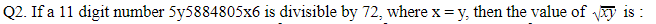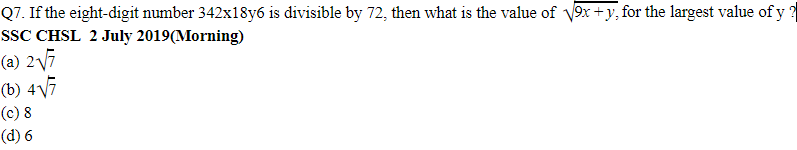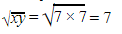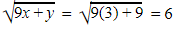# Number System: SSC CGL CHSL Math Questions

Validity: 9 Months
What you will get
Course Highlights
• Based on latest Pattern
• English Medium eBooks
Click to Bookmark
SSC

## Number System Math Previous Year Questions

In this, we are providing previous year questions of SSC CGL CHSL CPO MTS of number system with solutions asked by TCS. It is helpful for upcoming exams of SSC, Railways, and other competitive exams conducted by TCS.

### SSC Number System CGL Maths Previous Year Questions

Q1. If a nine-digit number 985x3678y is divisible by 72, then the value of (4x - 3y) is :

SSC CGL 4 June 2019 (Morning)

(a) 5

(b) 4

(c) 6

(d) 3SSC CGL 10 June 2019 (Morning)Q3. If a 10 digit number 2094x843y2 is divisible by 88, then the value of ( 5x - 7y ) for the largest possible value of x, is

SSC CGL 6 June 2019 (Evening)

(a) 3

(b) 5

(c) 2

(d) 6

Q4. What is the least value of x such that 517x324 is divisible by 12?

SSC CGL 11 June 2019 (Morning)

(a) 3

(b) 1

(c) 0

(d) 2

Q5. When an integer n is divided by 8, the remainder is 3. What will be the remainder if 6n-1 is divided by 8?

SSC CGL 13 June 2019 (Evening)

(a)4

(b)1

(c)0

(d)2

Q6. If a nine-digit number 43x1145y2 is divisible by 88, then the value of (3x-2y), for the smallest value of y, is:

SSC CHSL 1 July 2019(Evening)

(a) 22

(b) 18

(c) 20

(d) 9Q8. The ten-digit number 2x600000y8 is exactly divisible by 24. If x≠ 0 and y ≠0, then the least value of (x + y) is

SSC CHSL 11 July 2019(Morning)

(a) 5

(b) 8

(c) 9

(d) 2

Q 9. On dividing a number by 38, the quotient is 24 and the remainder is 13, then the number is:

SSC CPO 16 March 2019 (Morning)

(a) 925

(b) 975

(c) 904

(d) 956

Q10. What is the sum of the digits of the least number, which when divided by 12, 16, and 54, leaves the same remainder 7 in each case and is also completely divisible by 13?

SSC CPO 12 March 2019 (Evening)

(a) 36

(b) 16

(c) 9

(d) 27

### Here are the solutions to the above questions in short methods. It will help you to save your precious time in the exam.

Sol 1.

Ans. (b)

Since, 985x3678y is divisible by 72 it must be divisible by 9 and 8 (coprime factors of 72) and y must be an even number. So the sum of digits of this number must be divisible by 9 and last three digits by 8. 9+8+5+x+3+6+7+8+y = 46 + x + y

x+y must be 8 as after 46 nearest multiple of 9 is 54.

Pairs for 8 = (0,8)(1,7), (2,6), (3,5), (4,4), (5,3)(6,2)(7,1)(8,0)

Only pair which satisfies these conditions is (4,4). So the required value is 4(4)-3(4) = 4

Sol 2.

Ans. (c)

Since 5y5884805x6  is divisible by 72, it must be divisible by 9 and 8 (coprime factors of 72). So the sum of digits of this number must be divisible by 9 and last three digits by 8.

5+y+5+8+8+4+8+0+5+x+6⇒49+x+y,

Possible values of x+y = 5, 14

For x+y = 5

Possible values of x,y = (1,4), (2,3), (3,2),(4,1)

For x+y = 14

Possible values of x,y = (5,9), (6,8),(7,7), (8,6),(9,5)

Among these values, the last three digits of the number are divisible by 8 only when x=3 or 7

But for x=3, y = 2…...(x≠y)

Clearly, the desired values of x and y are 7 and 7 respectively.Sol 3.

Ans. (b)

2094x843y2 is divisible by 88, it must be divisible by 11 and 8(coprime factors of 88).

So, (2+9+x+4+y)-(0+4+8+3+2) = 0 or 11

x+y-2 = 0 or 11

For x+y-2 = 0

x+y = 2

Possible values of x,y = (0,2),(1,1),(2,0)

For x+y-2 = 11

x+y = 13

Possible values of x,y = (4,9), (5,8), (6,7), (7,6), (8,5), (9,4)

Last three digits of the number are divisible by 8 only for y = 1,5, 9

For y=1,9 (5x-7y) gives negative results which are not given in the option.

So the desired values of x and y are 8 and 5 respectively.

(5x-7y)5(8)-7(5) = 5 ans

Sol 4.

Ans. (d)

Since, 517x324 is divisible by 12 it must be divisible by 3 and 4(coprime factors of 12).

For a number to be divisible by 3 sum of its digits must be divisible by 3.

So, 5+1+7+x+3+2+4⇒ 22+x must be divisible by 3

Possible values of x = 2,5,8

Cleary the smallest value of x = 2

Sol 5.

Ans. (b)

Let the quotient is x.

So, n = 8x+3

6n-16(8x+3)-1

= 48x+17

48 is a multiple of 8 so 48 will be exactly divisible by 8. But when we divide 17 by 8 the remainder is 1. Ans

Alternate

choose the smallest value of n for which remainder is 3 when the number is divided by 8.

Let n = 11

6n-1 = 6(11)-1 = 65

Remainder when 65 is divided by 8 = 1

Sol 6.

Ans. (a)

Since, the number 43x1145y2 is divisible by 88, it must be divisible by 11 and 8.

For the number to be divisible by 11,

[(4+x+1+5+2)-(3+1+4+y) = 0 or 11

So, 4+x-y = 0 or 11

4+x-y =0 is possible for x=0,1,2,3,4,5 and y=5,6,7,8,9

4+x-y = 11 is possible for x = 7,8,9 and y = 0,1,2

Also a number is divisible by 8 if its last three digits are divisible by 8.

We will check for a minimum value of y

For y=0, the last three digits of the number are not divisible by 8.

For y = 1, the last three digits are 512 which is divisible by 8.

So the desired values of x and y are 8 and 1 respectively.

(3x - 2y) = 3(8)-2(1) = 22 ans

Sol 7.

Ans. (d)

Since the number 342x18y6 is divisible by 72, it must be divisible by 9 and 8.

We know that the sum of the digits of a number must be divisible by 9 if the number is divisible by 9.

Clearly, 3+4+2+x+1+8+y+6 ⇒24+x+y must be a multiple of 9.

Possible values of x+y = 3, 12 …….[45>(24+x+y)>24, as values of x and y, can’t be more than 9]

Also a number is divisible by 8 if its last three digits are divisible by 8.

We will check for a maximum value of y

For x+y = 12

( x,y ) = (3,9),(4,8),(5,7), (6,6), (7,5), (8,4),(9,3)

For y=9, the last three digits of the number are 896 which is divisible by 8.

So the values of x and y are 3 and 9 respectively.Sol 8.

Ans. (a)

Since 2x600000y8 is exactly divisible by 24, it must be divisible by 3 and 8.

We know that the sum of the digits of a number must be divisible by 3 if the number is divisible by 3.

Clearly, 2+x+6+y+8⇒ (16+x+y )must be the multiple of 3.

Possible values of x+y = 2,5,8,11,14 [(16+x+y) >16]

Numbers more than 9 get automatically eliminated as not given in the options.

So x+y must be 2,5 or 8.

Also a number is divisible by 8 if its last three digits are divisible by 8.

For x+y = 2

x=y=1 ...(xand y is not equal to zero)

Last three digits = 018 clearly it is not divisible by 8.

For x+y=5

x,y = (1,4), (2,3), (3,2) and (4,1)

For y=3,2,1 last three digits of the number are not divisible by 8 so these three values get eliminated.

For y=4 last three digits are 048 which is divisible by 8. Hence x=1 and y=4 are the desired values.

Sol 9.

Ans. (a)

Required number  = Divisor x Quotient + Remainder

= 38 x 24 + 13 = 925

Sol 10.

Ans. (b)

LCM of (12, 16 and 54) = 432

Let the number be (432k + 7)

ATQ: For (432k+7) to be exactly divisible by 13. {429k+(3k+7)} should also be divisible by 13.

Putting the value of k=1,2,3,... in (3k+7), k=2 satisfies the equation.

Therefore, Least possible number = 871

Sum of digits = 8+7+1 = 16